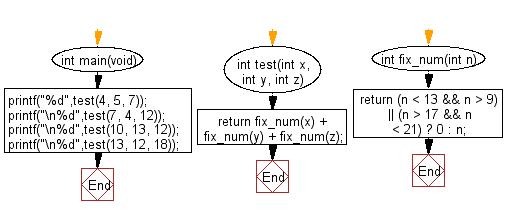﻿ C : Sum of the three integers will be 0, except for 13,17# C Exercises: Compute the sum of the three given integers. However, if any of the values is in the range 10..20 inclusive then that value counts as 0, except 13 and 17

## C-programming basic algorithm: Exercise-31 with Solution

Write a C program to compute the sum of the three given integers. However, if any of the values is in the range 10..20 inclusive then that value counts as 0, except 13 and 17.

C Code:

``````#include <stdio.h>
#include <stdlib.h>
int main(void){
printf("%d",test(4, 5, 7));
printf("\n%d",test(7, 4, 12));
printf("\n%d",test(10, 13, 12));
printf("\n%d",test(13, 12, 18));
}
int test(int x, int y, int z)
{
return fix_num(x) + fix_num(y) + fix_num(z);
}
int fix_num(int n)
{
return (n < 13 && n > 9) || (n > 17 && n < 21) ? 0 : n;
}
``````

Sample Output:

```16
11
13
13
```

Pictorial Presentation:Flowchart:C Programming Code Editor:

What is the difficulty level of this exercise?

Test your Programming skills with w3resource's quiz.

﻿

## C Programming: Tips of the Day

Why doesn't a+++++b work?

printf("%d",a+++++b); is interpreted as (a++)++ + b according to the Maximal Munch Rule!.

++ (postfix) doesn't evaluate to an lvalue but it requires its operand to be an lvalue.

! 6.4/4 says the next preprocessing token is the longest sequence of characters that could constitute a preprocessing token"

Ref : https://bit.ly/3fdldUT